#There are lots of suggestions for activities below to help you prepare for the Mathematics Key Stage 2 Tests (sometimes known as SATs). Click the images to go to the activities and tick the check boxes when you are confident with a topic to make it disappear! If you need more practice to read, write, order and compare numbers up to 10 000 000 and determine the value of each digit then click an icon below. If you need more practice to round any whole number to a required degree of accuracy then click an icon below. If you need more practice to use negative numbers in context, and calculate intervals across zero then click an icon below. If you need more practice to solve number and practical problems that involve other recently learnt mathematical skills then click an icon below. If you need more practice to multiply multi-digit numbers up to 4 digits by a two-digit whole number using the formal written method of long multiplication then click an icon below. If you need more practice to divide numbers up to 4 digits by a two-digit whole number using the formal written method of long division, and interpret remainders as whole number remainders, fractions, or by rounding, as appropriate for the context then click an icon below. If you need more practice to divide numbers up to 4 digits by a two-digit number using the formal written method of short division where appropriate, interpreting remainders according to the context then click an icon below. If you need more practice to perform mental calculations, including with mixed operations and large numbers then click an icon below. If you need more practice to identify common factors, common multiples and prime numbers then click an icon below. If you need more practice to use their knowledge of the order of operations to carry out calculations involving the four operations then click an icon below. If you need more practice to solve addition and subtraction multi-step problems in contexts, deciding which operations and methods to use and why then click an icon below. If you need more practice to solve problems involving addition, subtraction, multiplication and division then click an icon below. If you need more practice to use estimation to check answers to calculations and determine, in the context of a problem, an appropriate degree of accuracy. then click an icon below. If you need more practice to use common factors to simplify fractions; use common multiples to express fractions in the same denomination then click an icon below. If you need more practice to compare and order fractions, including fractions > 1 then click an icon below. If you need more practice to add and subtract fractions with different denominators and mixed numbers, using the concept of equivalent fractions then click an icon below. If you need more practice to multiply simple pairs of proper fractions, writing the answer in its simplest form then click an icon below.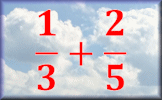If you need more practice to divide proper fractions by whole numbers then click an icon below.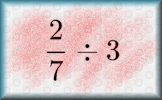If you need more practice to associate a fraction with division and calculate decimal fraction equivalents [for example, 0.375] for a simple fraction then click an icon below.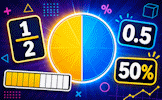If you need more practice to identify the value of each digit in numbers given to three decimal places and multiply and divide numbers by 10, 100 and 1000 giving answers up to three decimal places then click an icon below. If you need more practice to multiply one-digit numbers with up to two decimal places by whole numbers then click an icon below. If you need more practice to use written division methods in cases where the answer has up to two decimal places then click an icon below.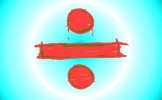If you need more practice to solve problems which require answers to be rounded to specified degrees of accuracy then click an icon below. If you need more practice to recall and use equivalences between simple fractions, decimals and percentages, including in different contexts. then click an icon below. If you need more practice to solve problems involving the relative sizes of two quantities where missing values can be found by using integer multiplication and division facts then click an icon below. If you need more practice to solve problems involving the calculation of percentages [for example, of measures, and such as 15% of 360] and the use of percentages for comparison then click an icon below. If you need more practice to solve problems involving similar shapes where the scale factor is known or can be found then click an icon below.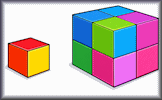If you need more practice to solve problems involving unequal sharing and grouping using knowledge of fractions and multiples. then click an icon below.If you need more practice to use simple formulae then click an icon below. If you need more practice to generate and describe linear number sequences then click an icon below. If you need more practice to express missing number problems algebraically then click an icon below. If you need more practice to find pairs of numbers that satisfy an equation with two unknowns then click an icon below. If you need more practice to enumerate possibilities of combinations of two variables. then click an icon below. If you need more practice to solve problems involving the calculation and conversion of units of measure, using decimal notation up to three decimal places where appropriate then click an icon below. If you need more practice to use, read, write and convert between standard units, converting measurements of length, mass, volume and time from a smaller unit of measure to a larger unit, and vice versa, using decimal notation to up to three decimal places then click an icon below. If you need more practice to convert between miles and kilometres then click an icon below.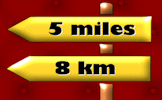If you need more practice to recognise that shapes with the same areas can have different perimeters and vice versa then click an icon below. If you need more practice to recognise when it is possible to use formulae for area and volume of shapes then click an icon below. If you need more practice to calculate the area of parallelograms and triangles then click an icon below. If you need more practice to calculate, estimate and compare volume of cubes and cuboids using standard units, including cubic centimetres and cubic metres, and extending to other units then click an icon below. If you need more practice to draw 2-D shapes using given dimensions and angles then click an icon below. If you need more practice to recognise, describe and build simple 3-D shapes, including making nets then click an icon below. If you need more practice to compare and classify geometric shapes based on their properties and sizes and find unknown angles in any triangles, quadrilaterals, and regular polygons then click an icon below. If you need more practice to illustrate and name parts of circles, including radius, diameter and circumference and know that the diameter is twice the radius then click an icon below.If you need more practice to recognise angles where they meet at a point, are on a straight line, or are vertically opposite, and find missing angles. then click an icon below. If you need more practice to describe positions on the full coordinate grid (all four quadrants) then click an icon below. If you need more practice to draw and translate simple shapes on the coordinate plane, and reflect them in the axes then click an icon below.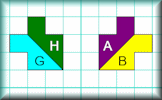If you need more practice to interpret and construct pie charts and line graphs and use these to solve problems then click an icon below. If you need more practice to calculate and interpret the mean as an average then click an icon below. Please note that the exercises and activities suggested in the links above are not always exact matches for the statements. Often they will be multi-levelled tasks containing content both below and above the standard suggested by the statement. If you are using a computer hover your cursor over the picture to see the details of the activity. Do you have any comments? It is always useful to receive feedback and helps make this free resource even more useful for those learning Mathematics anywhere in the world. Click here to enter your comments.For All: